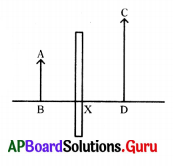Practice the AP 9th Class Physical Science Bits with Answers Chapter 7 Reflection of Light at Curved Surfaces on a regular basis so that you can attempt exams with utmost confidence.

## AP State Syllabus 9th Class Physical Science Bits 7th Lesson Reflection of Light at Curved Surfaces with Answers

Question 1.
Magnification m = …………………
A) $$\frac{\mathrm{v}}{\mathrm{u}}$$
B) $$\frac{\mathrm{u}}{\mathrm{v}}$$
C) $$\frac{\mathrm{h}_{\mathrm{o}}}{\mathrm{h}_{\mathrm{i}}}$$
D) $$\frac{\mathrm{h}_{\mathrm{i}}}{\mathrm{h}_{\mathrm{o}}}$$
D) $$\frac{\mathrm{h}_{\mathrm{i}}}{\mathrm{h}_{\mathrm{o}}}$$

Question 2.
A ray which seems to be travelling through the focus of a convex mirror passes ……………….. after reflection.
A) parallel to the axis
B) along the same path in opposite direction
C) through F
D) through C
A) parallel to the axisQuestion 3.
Magnification of a convex mirror is
A) = 1
B) < 1 C) > 1
D) All of the above
B) < 1

Question 4.
Dentist uses ……………… to observe teeth.
A) concave mirror
B) concave lens
C) convex mirror
D) plane mirror
A) concave mirror

Question 5.
The magnification of an object placed at a distance of 6 cm in front of a concave mirror is -3. It means ……………………..
A) The image is formed at a distance of 2 cm in front of the mirror
B) The image is formed at a distance of 2 cm in the mirror
C) The image is formed at a distance of 18 cm in front of the mirror
D) The image is formed at a distance of 18 cm in the mirror
C) The image is formed at a distance of 18 cm in front of the mirror

Question 6.
Where should we keep the object to get a large, virtual image with concave mirror ?
A) at ‘F’
B) at ‘C’
C) beyond ‘C’
D) between F and P
D) between F and P

Question 7.
The mirror which is bent inwards is called …………………. mirror.
A) Plane
B) Concave
C) Convex
D) None of these
B) Concave

Question 8.
The mirror which is bent outwards is called ………………. mirror.
A) Concave
B) Convex
C) Plane
D) None of these
B) Convex

Question 9.
Concave mirror is part of …………………
A) Cone
B) Cylinder
C) Cuboid
D) Sphere
D) SphereQuestion 10.
The centre of mirror is called ……………………
A) Centre of curvature
B) Vertex
D) Focus
B) Vertex

Question 11.
The rays coming from object parallel to concave mirror will converge at …………………..
A) Focus
B) Vertex
C) Centre of curvature
A) Focus

Question 12.
When object is placed between mirror and focus, the image is …………………….
A) Same size
B) Diminished
C) Highly diminished
D) Enlarged
D) Enlarged

Question 13.
When an object is placed on centre of curvature the size of image is ………………..
A) Same size
B) Diminished
C) Highly diminished
D) Enlarged
A) Same size

Question 14.
For a concave mirror, the focal length is ………………….
A) Positive
B) Negative
C) Zero
D) None of these
A) Positive

Question 15.
The scientist who used mirrors to burn ships was ………………..
A) Newton
B) Fermat
C) Archimedes
D) Pascal
C) Archimedes

Question 16.
……………… mirror is used in solar cookers.
A) Plane
B) Convex
C) Concave
D) None of these
C) ConcaveQuestion 17.
The light ray which passes through ………………. will reflect in the same path from a spherical mirror.
A) Focus
B) Vertex
C) Centre of curvature
D) None of these
C) Centre of curvature

Question 18.
The rear view mirror used in vehicles is
A) Plane
B) Convex
C) Concave
D) None of these
B) Convex

Question 19.
A radius drawn from the centre of the circle to point on the circle will be ………………… to the tangent to that point on the circle.
A) parallel
B) perpendicular
C) equal
D) none of these
B) perpendicular

Question 20.
Radius of curvature = …………… × focal length
A) 3
B) 4
C) 2
D) 5
C) 2

Question 21.
The radius of curvature of a concave mirror is 30 cm. If the object placed at 10 cm from the mirror, then the image is
A) Magnified real image
B) Diminished real image
C) Diminished virtual image
D) Magnified virtual image
D) Magnified virtual image

Question 22.
Which of the following images cannot be caught on the screen ?
A) Real
B) Virtual
C) Lateral inversion
D) None of these
B) Virtual

Question 23.
Which of the following mirrors is used by dentists ?
A) Concave
B) Convex
C) Bi-concave
D) Bi-convex
A) ConcaveQuestion 24.
Mirror formula
A) $$\frac{1}{\mathrm{f}}=\frac{1}{\mathrm{u}}+\frac{1}{\mathrm{v}}$$
B) $$\frac{1}{\mathrm{f}}=\frac{1}{\mathrm{u}}-\frac{1}{\mathrm{v}}$$
C) $$\frac{1}{\mathrm{f}}=\frac{\mathrm{v}}{\mathrm{u}}$$
D) $$\frac{1}{\mathrm{f}}=-\frac{\mathrm{u}}{\mathrm{v}}$$
A) $$\frac{1}{\mathrm{f}}=\frac{1}{\mathrm{u}}+\frac{1}{\mathrm{v}}$$

Question 25.
When parallel rays are incident on a concave mirror, on reflection they meet at the ……………….
A) Pole
B) Centre of curvature
C) Focus
D) None of these
C) Focus

Question 26.
Which of the following is the formula of magnification ?
A) $$\frac{\mathrm{v}}{\mathrm{u}}$$
B) $$\frac{\mathrm{u}}{\mathrm{v}}$$
C) $$\frac{\mathrm{v}}{-\mathrm{u}}$$
D) $$\frac{-\mathrm{u}}{\mathrm{v}}$$
B) $$\frac{\mathrm{u}}{\mathrm{v}}$$

Question 27.
Where is the image formed, when the object is placed between mirror and focus ?
A) Behind the mirror
B) At infinity
C) Beyond C
D) On C
A) Behind the mirror

Question 28.
Which type of mirrors are used in headlights of the vehicles ?
A) Concave
B) Convex
C) Bi-concave
D) Bi-convex
A) Concave

Question 29.
Which mirrors are used in many vehicles as rear view mirrors and window glasses of a car ?
A) Concave
B) Convex
C) Bi-concave
D) Bi-convex
B) Convex

Question 30.
For which mirrors the magnification is always same ?
A) Concave mirror
B) Convex mirror
C) Plane mirror
D) All
C) Plane mirrorQuestion 31.
The shaving mirror, dentist’s mirror work on, when the object is …………………..
A) at centre of curvature
B) between centre of curvature and focus
C) at focus or focal point
D) between focus and pole
D) between focus and pole

Question 32.
The angle of incidence is the angle between
A) The incident ray and surface of the mirror
B) The reflected ray and surface of the mirror
C) The normal to the surface and incident ray
D) The normal to the surface and reflected ray
C) The normal to the surface and incident ray

Question 33.
f = $$\frac{\mathbf{R}}{\mathbf{2}}$$ is valid
A) For convex mirrors only
B) For concave mirrors only
C) Both convex and concave mirrors
D) Neither convex nor concave mirrors
C) Both convex and concave mirrors

Question 34.
A ray of light is incident on a concave mirror. If it is parallel to the principal axis, the reflected ray will ………………..
A) Pass through the focus
B) Pass through centre of curvature
C) Pass through pole
D) Retrace its path
A) Pass through the focus

Question 35.
If an incident ray passes through the centre of curvature of a spherical mirror, the reflected ray will ………………….
A) Pass through focus
B) Pass through centre of curvature
C) Pass through pole
D) Retrace its path
D) Retrace its path

Question 36.
A diverging mirror is …………………
A) A plane mirror
B) Convex mirror
C) Concave mirror
D) A shaving mirror
B) Convex mirror

Question 37.
Light always selects the path which takes the least time to travel. This was first given by ………………..
A) Fermat
B) Newton
C) Robert Hook
D) Huygen’s
A) FermatQuestion 38.
The magnification of mirror is negative, the image is
A) Smaller than the object
B) Larger than the object
C) Erect
D) Inverted
D) Inverted

Question 39.
No matter how far you stand from a mirror, your image appears erect. The mirror is likely to be
A) Concave only
B) Plane only
C) Convex only
D) Either plane at Convex
D) Either plane at Convex

Question 40.
In the following which is the formula for magnification for mirror ?
A) m = $$\frac{\mathrm{v}}{\mathrm{u}}$$
B) m = $$\frac{\mathrm{h}_{\mathrm{i}}}{\mathrm{h}_{\mathrm{o}}}$$
C) m = $$\frac{-\mathrm{v}}{\mathrm{u}}$$
D) Both B and C
D) Both B and C

Question 41.
The image of an object formed by a plane mirror is ………………………..
A) virtual
B) real
C) diminished
D) upside-down
A) virtual

Question 42.
Magnification produced by a convex mirror is always ……………………..
A) more than 1
B) less than 1
C) equal to 1
D) more or less than 1
B) less than 1

Question 43.
In order to obtain a magnification of ‘-2’ with a concave mirror the object should be placed ……………………
A) between pole and focus
B) between focus and centre of curvature
C) at the centre of curvature
D) beyond the centre of curvature
B) between focus and centre of curvature

Question 44.
Focal length of plane mirror is ………………………
A) zero
B) infinity
C) negative
D) positive
B) infinityQuestion 45.
In case of concave mirror, the minimum distance between a real object and its real image is …………………..
A) f
B) 2f
C) 4f
D) zero
D) zero

Question 46.
A real, inverted and equal in size image is formed by ……………….
A) A plane mirror
B) A convex mirror
C) A concave mirror
D) A spherical mirror
C) A concave mirror

Question 47.
A ray of light is incident on a concave mirror if it is parallel to the principal axis, the reflected ray will ………………………
A) retrace its path
B) pass through the pole
C) pass through the centre of curvature
D) pass through the focus
D) pass through the focus

Question 48.
The image formed by a concave mirror is virtual, erect and magnified. The position of object is ………………….
A) at focus
B) between focus and centre of curvature
C) at pole
D) between pole and focus
D) between pole and focus

Question 49.
The radius of curvature and focal length of a concave mirror are ……………………
A) zero
B) negative
C) positive
D) infinity
B) negative

Question 50.
Match the following.

 Column – I Column – II 1. Concave a) Rear-view mirror in vehicles 2. Convex mirror always b) Virtual images 3. Plane mirror c) Light passes through 4. Glass plate d) Real and virtual images

A) 1 → a, 2 → b, 3 → c, 4 → d
B) 1 → b, 2 → c, 3 → d, 4 → a
C) 1 → c, 2 → d, 3 → a, 4 → b
D) 1 → d, 2 → a, 3 → b, 4 → c
D) 1 → d, 2 → a, 3 → b, 4 → c

Question 51.
A mirror forms a virtual image of a real object ………………..
A) It must be a concave mirror
B) It must be a plane mirror
C) It must be a convex mirror
D) It may be any of the mirrors mentioned above.
D) It may be any of the mirrors mentioned above.Question 52.
The real image formed by a concave mirror is larger than the object when the object is ………………………..
A) at a distance equal to radius of curvature
B) at a distance less than the focal length
C) between focus and centre of curvature
D) at a distance greater than radius of curvature
C) between focus and centre of curvature

Question 53.
The mirror which can form a magnified image of an object is ……………………
A) convex mirror
B) plane mirror
C) concave mirror
D) both convex and concave mirrors
C) concave mirror

Question 54.
All the distances in case of spherical mirror are measured in relation to …………………….
A) The focus of the mirror
B) The image to the object
C) Object to image
D) The pole of the mirror
D) The pole of the mirror

Question 55.
In a convex spherical mirror, reflection of light takes place at ………………….
A) a flat surface
B) a bent-in surface
C) a bulging out surface
D) an uneven surface
C) a bulging out surface

Question 56.
The minimum distance of an object from concave mirror for which the mirror forms real image is (let T be the focal length of concave mirror)
A) f
B) 2f
C) 4f
D) zero
A) f

Question 57.
The focal length of a spherical mirror of radius of curvature 30 cm is …………………..
A) 10 cm
B) 15 cm
C) 20 cm
D) 30 cm
B) 15 cm

Question 58.
The image formed by a concave mirror is observed to be virtual, erect and larger than the object. Where should be the position of the object ?
A) Between the pole of the mirror and its principal focus
B) Beyond the centre of curvature
C) At the centre of curvature
D) Between the principal focus and the centre of curvature
A) Between the pole of the mirror and its principal focusQuestion 59.
The properties of image when a real object is kept in between focus and centre of curvature of a concave mirror ……………………
A) Size of image is greater than size of object
B) Image is inverted
C) Image is real
D) All the above
D) All the above

Question 60.
A real image
A) is formed when reflected rays do not actually meet but appear to diverge from a point
B) can’t be obtained on a screen
C) can be obtained on a screen
D) is always erect
C) can be obtained on a screen

Question 61.
The image of a bright object is brought on a screen with the help of a concave mirror. If the upper half of the concave mirror is covered, the effect on the image will be
A) its size becomes half
B) image disappears
C) brightness reduced
D) image changes position
C) brightness reduced

Question 62.
The relation between the radius of curvature ‘R’ and focal length of a concave mirror is
A) f = 2R
B) f = R
C) $$\frac{\mathbf{R}}{\mathbf{2}}$$
D) $$\frac{\mathbf{R}}{\mathbf{4}}$$
C) $$\frac{\mathbf{R}}{\mathbf{2}}$$

Question 63.
A ray of light strikes the concave mirror parallel to principal axis. After reflection from the mirror
A) it passes to the centre of curvature of the mirror
B) it passes through the focus of the mirror
C) it is sent back along the same direction
D) none of the above options
B) it passes through the focus of the mirror

Question 64.
An object of size 2.5 cm is kept perpendicular to the principal axis of a concave mirror. The distance of the object from the pole of the mirror equals the radius of curvature of mirror. The size of the image formed is
A) 5.0 cm
B) 3.5 cm
C) 2.5 cm
D) 1.25 cm
C) 2.5 cm

Question 65.
An object is kept at the centre of curvature of a concave mirror, the distance between the pole of the mirror and the image
A) equal to ‘f’
B) between ‘f’ and ‘2f’
C) greater than 2f
D) equal to ‘2f’
D) equal to ‘2f’Question 66.
For doing the experiment it is advisable to use a piece of wood or hard cardboard in the place of paper. Because
A) Paper is costlier
B) White sheet of paper is not easily available
C) When sun rays from a distant object are focused on a sheet of paper, it may start burning
D) None of the above reasons
C) When sun rays from a distant object are focused on a sheet of paper, it may start burning

Question 67.
The relation between u, v and f for a spherical mirror using New Cartesian convention is
A) v – u = f
B) $$\frac{1}{v}-\frac{1}{u}=\frac{1}{f}$$
C) $$\frac{1}{v}+\frac{1}{u}=\frac{1}{f}$$
D) v + u = f
C) $$\frac{1}{v}+\frac{1}{u}=\frac{1}{f}$$

Question 68.
Image formed by a convex mirror is always,
A) real and magnified
B) real and small
C) virtual and magnified
D) virtual and diminished
D) virtual and diminished

Question 69.
A virtual, erect and magnified image is formed by a concave mirror when the object is
A) at focus
B) between focus and centre of curvature
C) beyond centre of curvature
D) between pole and focus
D) between pole and focus

Question 70.
S.I unit of linear magnification is
A) m
B) m-1
C) m2
D) no unit
D) no unit

Question 71.
The mirror which has a wider field of view is
A) convex mirror
B) concave mirror
C) plane mirror
D) none of above
A) convex mirror

Question 72.
The imaginary line passing through the centre of curvature and the pole of the mirror is called
A) principal axis
C) focal plane
D) focal length
A) principal axisQuestion 73.
A mirror which forms a virtual image only for some particular position of the object with respect to the mirror is a
A) plane mirror
B) convex mirror
C) concave mirror
D) none of above
C) concave mirror

Question 74.
A strip is cut from a hollow glass sphere and is treated from outside so that reflection of light takes place from hollow inner side, such a mirror is called a
A) plane mirror
B) convex mirror
C) concave mirror
D) none of above
C) concave mirror

Question 75.
The distance between the two opposite points on circular boundary of a mirror is called its
A) focal length
C) aperture
D) principal axis
C) aperture

Question 76.
A strip is cut from a hollow glass sphere and hollow inner surface is treated in such a way that the reflection of light takes place from the outer surface. Such a mirror is called a
A) convex mirror
B) concave mirror
C) plane mirror
D) none of above
A) convex mirror

Question 77.
The image of a distant object is obtained on a screen by using a concave mirror. The focal length of the concave mirror can be determined by measuring the distance between
A) the object and the screen
B) the mirror and the screen
C) the object and the mirror
D) A & B both
B) the mirror and the screen

Question 78.
A student obtained a blurred image of an object on a screen by using a concave mirror. In order to obtain a sharp image on the screen, he will have to shift the mirror
A) towards the screen
B) away from the screen
C) far away from the screen
D) either away from or towards, the screen depending upon the position of object
D) either away from or towards, the screen depending upon the position of object

Question 79.
In solar devices, the energy is concentrated into a small area using
A) plane mirror
B) concave mirror
C) parabolic mirror
D) parabolic or concave mirror
D) parabolic or concave mirrorQuestion 80.
From which point all the distances are measured in case of a concave mirror?
A) pole
B) centre of curvature
C) focus
D) any of above
A) pole

Question 81.
Parallel rays from a distant pole incident on a concave mirror form a sharp image on the screen placed at the focus of the mirror the image will be
A) real & inverted
B) real & erect
C) virtual & inverted
D) virtual & erect
A) real & inverted

Question 82.
For positive magnification produced by a concave mirror, the position of the object should be
A) between pole and focus
B) at the centre of curvature
C) at the focus
D) at infinity
A) between pole and focus

Question 83.
The point at which principal axis is perpendicular to the reflecting surface of concave mirror is called
A) centre of curvature
B) focus
C) pole
D) none of the above
C) pole

Question 84.
In case of concave mirror magnification for the real image is
A) negative
B) positive
C) neither negative nor positive
D) either positive or negative
A) negative

Question 85.
The position of the object for which magnification produced by concave mirror is 1, will be,
A) at focus
B) between focus and pole
C) centre of curvature
D) infinity
C) centre of curvature

Question 86.
The image formed by a concave mirror is real. The position of the screen should be
A) on the same side of the object between focus and infinity
B) behind the mirror
C) on the same side of the object between pole and focus
D) any of above three
A) on the same side of the object between focus and infinityQuestion 87.
Assertion (A) : Light forms shadow.
Reason (R) : Light always travels in a straight line.
A) ‘A’ and ‘R’ are correct and ‘A’ is supported by ‘R’
B) ‘A’ and ‘R’ are correct, but ‘A’ is not supported by ‘R’
C) ‘A’ is correct, but ‘R’ is incorrect
D) ‘R’ is correct, but ‘A’ is incorrect
A) ‘A’ and ‘R’ are correct and ‘A’ is supported by ‘R’

Question 88.
Assertion (A1) :When parallel rays are incident on a concave mirror, on reflection they meet at the focus.
Assertion (A2 :We get an image with a concave mirror at the focus every time.
A) A1 is correct, but A2 is wrong.
B) A1 is wrong, but A2 is correct.
C) Both A1 and A2 are correct.
D) Both A1 and A2 are wrong.
A) A1 is correct, but A2 is wrong.

Question 89.
Assertion (A) rDentists use convex mirrors.
Reason (R) : Convex mirrors enlarge the image when the object is held close to the mirror.
A) ‘A’ is correct, but ‘R’ is wrong.
B) ‘A’ is wrong, but ‘R’ is correct.
C) Both ‘A’ and ‘R’ are correct.
D) Both ‘A’ and ‘R’ are wrong.
D) Both ‘A’ and ‘R’ are wrong.

Question 90.
If an object is placed at C on the principal axis in front of a concave mirror, the position of the image is ……………..
A) at infinity
B) between F and C
C) at C
D) beyond C
C) at C

Question 91.
We get a diminished image with a concave mirror when the object is placed …………………
A) at F
B) between the pole and F
C) at C
D) beyond C
D) beyond C

Question 92.
We get a virtual image in a concave mirror when the object is placed …………………
A) at F
B) between the pole and F
C) at C
D) beyond C
B) between the pole and F

Question 93.
A teacher held a pencil close to a spherical mirror and asked four students W, X, Y and Z to predict the nature of the mirror with the help of the image formed in the mirror. The image was erect and enlarged.
The four students identified it as follows :
W – Convex in nature
X – Concave in nature
Y – Plane mirror
Z – Plano-concave mirror
The correct statement was given by ……………….
A) W
B) X
C) Y
D) Z
B) XQuestion 94.
We get a virtual image with a concave mirror when the object is placed between
A) vertex and focus
B) focus and centre of curvature
C) on centre of curvature
D) beyond centre of curvature
A) vertex and focus

Question 95.
No matter how far you stand from a mirror, your image appears erect. The mirror is likely to be
A) Plane
B) Concave
C) Convex
D) Either plane or convex
D) Either plane or convex

Question 96.
The magnification is always 1 for the following mirror
A) Convex mirror
B) Concave mirror
C) Plane mirror
D) None of these
C) Plane mirror

Question 97.
The mirror for which the magnification is always less than 1.
A) convex mirror
B) concave mirror
C) plane mirror
D) none of these
A) convex mirror

Question 98.
The image formed due to a concave mirror is highly enlarged with the object placed
A) At pole
B) Beyond centre of curvature
C) At centre of curvature
D) At focus
D) At focus

Question 99.
A concave mirror forms a point sized image for a object at
A) Focus
B) Infinity
C) Beyond centre of curvature
D) Centre of curvature
B) Infinity

Question 100.
The mirror which always give diminished image is
A) Plane mirror
B) Convex mirror
C) Concave mirror
D) None of these
B) Convex mirrorQuestion 101.
Which of the following mirrors is used by Archimedes to burn the ships?
A) Bi-concave
B) Bi-convex
C) Concave
D) Convex
C) Concave

Question 102.
Where is the object placed, on principal axis of a concave mirror to get the image at infinity ?
A) Between P and F
B) On focal point
C) Between F and C
D) Beyond C
B) On focal point

Question 103.
Where is the object placed to get a virtual image in front of concave mirror?
A) Between mirror and F
B) On focal point
C) Between F and C
D) On C
A) Between mirror and F

Question 104.
Where is the object placed to get a same size of image and is inverted ?
A) Between P and F
B) On F
C) Between F and C
D) On C
D) On C

Question 105.
When parallel rays are incident on a concave mirror on reflection they meet at the
A) Pole
C) Focus
D) None of these
C) Focus

Question 106.
A concave mirror has a focal length of 25 cm. A person wants to see his face in this mirror. At what distance should the person stand ?
A) 40 cm
B) 30 cm
C) 20 cm
D) 25 cm
C) 20 cm

Question 107.
A student observed the image of an object placed in front of a plane mirror. He wrote the properties of the image of an object. Which of the following is wrong ?
A) Image is virtual and erect.
B) The size of the image is equal to size of the object.
C) Image is laterally inverted.
D) Distance of the image behind the mirror is greater than the distance of the object from the mirror.
D) Distance of the image behind the mirror is greater than the distance of the object from the mirror.Question 108.
To get an image larger than the object, one can use
A) Convex mirror
B) Concave mirror
C) Plane mirror
D) Both convex and concave mirrors
B) Concave mirror

Question 109.
If the magnification of a mirror is greater than 1, then the mirror is
A) Concave mirror
B) Convex mirror
C) Plane mirror
D) None of the above
A) Concave mirror

Question 110.
Magnification has a plus sign, then the image is ………………….
A) Virtual and erect
B) Real and erect
C) Real and inverted
D) At infinity
A) Virtual and erect

Question 111.
What type of image is formed on a cinema screen ?
A) virtual
B) real
C) both types
D) none of the above
B) real

Question 112.
A real object is placed between pole and principal focus of a convex mirror. The image produced is
A) virtual, diminished and inverted
B) virtual, magnified and upright
C) virtual, diminished and upright
D) real, diminished and inverted
B) virtual, magnified and upright

Question 113.
Mirror used by dental surgeon is
A) concave
B) convex
C) plane
D) both concave and convex
A) concave

Question 114.
Which of the following mirrors is used in search light reflector ?
A) concave
B) convex
C) plane
D) any of above
A) concaveQuestion 115.
Your school laboratory has one large window, to find the focal length of a concave mirror using one of the walls as the screen, the experiment may be formed
A) near the wall opposite to the window
B) on the same wall as the window
C) on the wall adjacent to the window
D) only on the table as per the laboratory arrangement
B) on the same wall as the window

Question 116.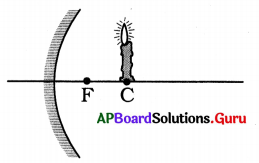Predict the image.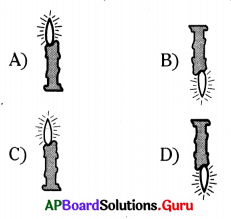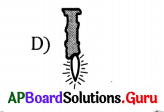Question 117.
Here 4 mirrors are given with same radius. Which one has the highest focal length among them if the focal length of the ‘A’ = 10 cm?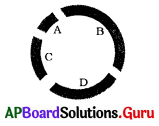A) D
B) B
C) C
D) fs’ of all are equal
D) fs’ of all are equal

Question 118.
A student experimented by using a concave mirror with focal length 10 cm. But accidentally, it slipped off his hands and is broken. He did experiment with larger piece of that mirror. The focal length obtained in his experiment is ………………..
A) 5 cm
B) 10 cm
C) 15 cm
D) 20 cm
B) 10 cm

Question 119.
A mirror always forms an erected, diminished and virtual image for an object placed at different positions. The observations noted by student are, as follows.
I) It is a concave mirror.
II) Magnification of that image is always less than one (m < 1).
III) The mirror can be used as rear view mirror.
The correct observations are
A) I, II
B) II, III
C) I, III
D) I, II, III
B) II, III

Question 120.
A concave mirror of focal length ‘f’ produces an image ‘n’ times the size of the object. If the image is real, then the distance’d’ of the object from the mirror is
A) $$\frac{(n+1) f}{n}$$
B) (n + 1) f
C) (n – 1) f
D) $$\frac{(n-1) f}{n}$$
A) $$\frac{(n+1) f}{n}$$

Question 121.
A spherical mirror and a thin spherical lens have each a focal length of – 15 cm. The mirror and the lens are likely to be ……………….
A) both are convex
B) both are concave
C) the mirror is convex but the lens are concave
D) the mirror is concave and the lens are convex
B) both are concaveQuestion 122.
A teacher held a pencil close to a spherical mirror and asked four students P, Q, R, S to predict the nature of the mirror with the help of the image formed in the mirror. The image was erect and enlarged. The four students identified it as follows :
P – convex in nature
Q – concave in nature
R – plane mirror
S – plane concave mirror
The correct statement is of ………………..
A) P
B) Q
C) R
D) S
B) Q

Question 123.
The angle of incidence between the plane of a mirror and light ray is 45°. The angle of reflection is …………………
A) 45°
B) 30°
C) 90°
D) 60°
A) 45°

Question 124.
The image of a distant object is obtained on a screen by using a concave mirror. The focal length of the mirror can be determined by measuring the distance between
A) The object and the screen
B) The object and the mirror
C) The mirror and the screen as that between the object and the screen
D) The mirror and the screen
D) The mirror and the screen

Question 125.
A ray of light is incident on a plane mirror making an angle of 90° with the mirror surface. The angle of reflection for this ray of light will be ………………….
A) 45°
B) 90°
C) 0°
D) 60°
C) 0°

Question 126.
To get an image larger than object one can use …………………
A) A concave mirror but not a convex mirror
B) A convex mirror but not a concave mirror
C) A plane mirror
D) Either a convex mirror or a concave mirror
A) A concave mirror but not a convex mirror

Question 127.
If the object is placed at focus of a concave mirror, the image is formed at ………………
A) focus
B) infinity
C) between ‘F and ‘O’
D) centre of curvature
B) infinity

Question 128.
Salmon has to perform the experiment of finding the focal length of a given concave mirror by using a distant object. Which of the following ‘setups’ will he choose apart from the mirror and screen provided ?
A) a mirror holder, and a scale
B) a mirror holder, a screen holder and a scale
C) a screen holder and a scale
D) a mirror holder and a screen holder
B) a mirror holder, a screen holder and a scaleQuestion 129.
Find the focal length of a spherical mirror when the radius of curvature is 20 cm.
A) 10 cm
B) 20 cm
C) 30 cm
D) 40 cm
A) 10 cm

Question 130.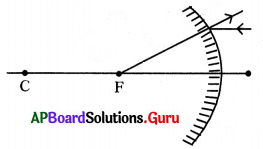This figure shows that
1. Mirror is convex.
2. Mirror is concave.
3. It forms virtual image.
4. It forms real image.
A) 1 and 2 correct
B) 1 and 3 correct
C) 2 and 3 correct
D) 2 and 4 correct
B) 1 and 3 correct

Question 131.

 Situation Mirror Place of an object 1. Convex Between F & mirror 2. Concave Between F & mirror 3. Concave Between F & C 4. Concave Beyond C 5. Convex Between F & C

In which situation inverted image is formed ?
A) 1
B) 2 & 5
C) 2, 3 & 4
D) 3 & 4
D) 3 & 4

Question 132.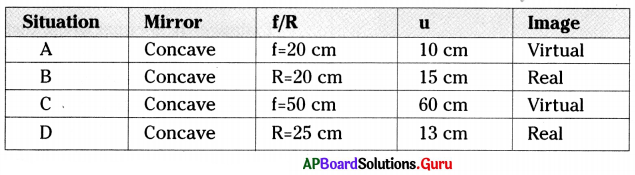Which situation does not occur ?
A) AA
B) BA
C) CA
D) D
C) CA

Question 133.

 Situation Mirror Magnification A Plane > 1 B Concave > 1 C Convex < 1 D Concave < 1

Which situation does not take place ?
A) A
B) B
C)C
D) D
A) A

Question 134.
Magnification of the image in the given figure is ………….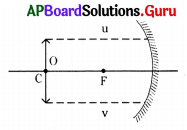A) M = -1
B) M = 1
C) M = 0
D) M > 1
A) M = -1

Question 135.
The angle of reflection in the given figure is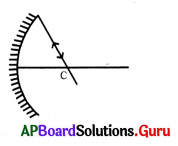A) 90°
B) 60°
C) 0°
D) 30°
C) 0°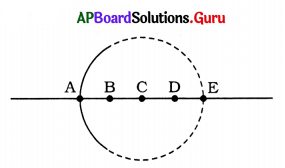Question 136.
Generally which part is not indicated on the axis for ray diagrams.
A) A
B) B
C) C
D) D
D) D

Question 137.
Correct mark of the focal point is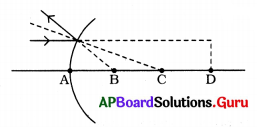A) A
B) B
C) C
D) D
B) B

Question 138.
Which one is the lable of the normal?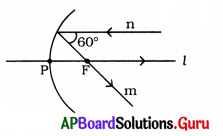A) n
B) m
C) l
D) not in the figure
C) l

Question 139.
Convex mirror is used as ………………..
A) Shaving mirror
B) Focusing mirror
C) Rear-view mirror
C) Rear-view mirror

Question 140.
The adjoining figure shows a concave mirror with its principal axis passing through points O, F and X.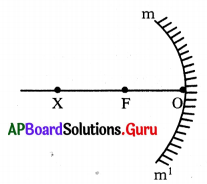D istance OF is equal to the distance FX = focal length ‘f’. The point X on the principal axis is called
A) focus of the mirror
B) optical centre of the mirror
C) centre of curvature of the mirror
D) none of the above
C) centre of curvature of the mirror

Question 141.
A ray of light PQ parallel, is incident at the point Q on the mirror as shown in the figure. The ray after suffering reflection from the mirror passes through the point.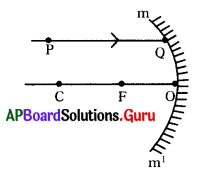A) C
B) F
C) that lies between ’O’ and ‘F’
D) that lies between C and F
B) F

Question 142.
In the experiment to find the focal length of a concave mirror, a graph is drawn between ‘V’ and ‘U’. The graph looks like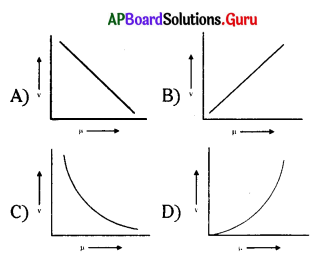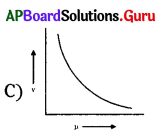Question 143.
Out of the following, the best way to do the experiment for finding the focal length of a concave mirror by obtaining the image of distant object is to
A) keep both the mirror and the screen in suitable stands with screen put in front of the mirror.
B) keep both the mirror and the screen in suitable stands with screen put behind the mirror.
C) hold the mirror in hand and keep the screen in a stand behind the mirror.
D) hold the mirror in a stand and hold the screen in hand infront of the mirror.
A) keep both the mirror and the screen in suitable stands with screen put in front of the mirror.

Question 144.
The mirror used by ENT specialists is
A) plane
B) concave
C) convex
D) none of these
B) concave

Question 145.
In torches, search-lights and head-lights of vehicles, the bulb is placed ………………
A) between the pole and the focus of the reflector.
B) at the focus of the reflector.
C) at the centre of curvature of the reflector.
D) between the focus and the centre of curvature of the reflector.
B) at the focus of the reflector.

Question 146.
Which of the following mirror is used as rear view mirror ?
A) Concave
B) Convex
C) Plane
D) Combination of concave and convex
B) Convex

Question 147.
When light gets reflected from a curved surface, if the angle of incidence is 30° then what about the angle of reflection ?
A) 150°
B) 30°
C) 45°
D) 60°
B) 30°

Question 148.
An object of size 7 cm is placed at 27 cm in front of a concave mirror of focal length 18 cm. Find the image distance.
A) 54 m
B) – 54 m
C) – 54 cm
D) 54 cm
C) – 54 cm

Question 149.
An object of size 7 cm is placed at 27 cm in front of a concave mirror of focal length (f) = 18 cm. Find the size of the image.
A) 21 cm
B) 21 m
C) – 21 cm
D) – 21 m
C) – 21 cm

Question 150.
What is the radius of curvature of mirror if focal length is 20 cm ?
A) 20 cm
B) 40 cm
C) 60 cm
D) 80 cm
B) 40 cmQuestion 151.
A concave mirror is made by cutting a portion of a hollow glass sphere of radius 24cm. Find the focal length of the mirror.
A) 12 cm
B) 18 cm
C) 24 cm
D) 6 cm
A) 12 cm

Question 152.
Looking into a mirror one finds his image long and thin; the mirror is ………………….
A) parabolic
B) concave
C) convex
D) cylindrical
D) cylindrical

Question 153.
Which of the following is not related to a concave mirror ?
A) Rearview mirror
B) Shaving mirror
D) TV dish antenna
A) Rearview mirror

Question 154.
The phenomenon of the following diagram is due to ……………… of light.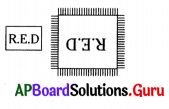A) Refraction
B) Reflection
C) T.I.R
D) Dispersion
B) Reflection

Question 155.
If the incident ray of light passes through the principal focus of a concave mirror as shown in the diagram, the reflected ray will ……………………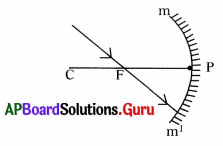A) pass through the pole
B) pass through the centre of curvature
C) be parallel to the principal axis
D) pass through the focus
C) be parallel to the principal axis

Question 156.
Which of the following diagrams depicts correctly the image formation of a distant object ?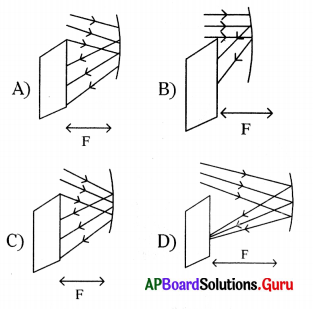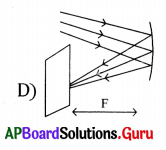Question 157.
A student focused the image of a distant object on the screen, as shown in the figure by using a device ‘X’. The device X could be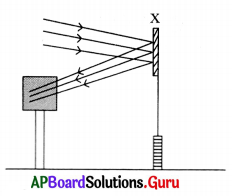A) plane mirror
B) concave mirror
C) convex mirror
D) convex lens